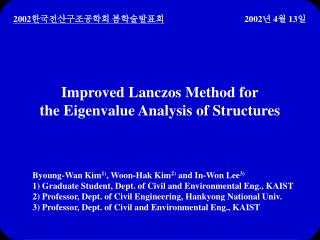DownloadDownload PresentationImproved Lanczos Method for the Eigenvalue Analysis of Structures

# Improved Lanczos Method for the Eigenvalue Analysis of Structures

Télécharger la présentation## Improved Lanczos Method for the Eigenvalue Analysis of Structures

- - - - - - - - - - - - - - - - - - - - - - - - - - - E N D - - - - - - - - - - - - - - - - - - - - - - - - - - -
##### Presentation Transcript

1. 2002한국전산구조공학회 봄학술발표회 2002년 4월 13일 Improved Lanczos Method for the Eigenvalue Analysis of Structures Byoung-Wan Kim1), Woon-Hak Kim2) and In-Won Lee3) 1) Graduate Student, Dept. of Civil and Environmental Eng., KAIST 2) Professor, Dept. of Civil Engineering, Hankyong National Univ. 3) Professor, Dept. of Civil and Environmental Eng., KAIST

2. Contents • Introduction • Matrix-powered Lanczos method • Numerical examples • Conclusions

3. Introduction • Background • Dynamic analysis of structures • - Direct integration method • - Mode superposition method  Eigenvalue analysis • Eigenvalue analysis • - Subspace iteration method • - Determinant search method • - Lanczos method • The Lanczos method is very efficient.

4. Literature review • The Lanczos method was first proposed in 1950. • Erricson and Ruhe (1980): • Lanczos algorithm with shifting • Smith et al. (1993): • Implicitly restarted Lanczos algorithm • Gambolati and Putti (1994): • Conjugate gradient scheme in Lanczos method

5. In the fields of quantum physics, Grosso et al. (1993) • modified Lanczos recursion to improve convergence.

6. Objective • Application of Lanczos method using the power technique • to the eigenproblem of structures in structural dynamics •  Matrix-powered Lanczos method

7. Matrix-powered Lanczos method • Eigenproblem of structure

8. Modified Gram-Schmidt process of Krylov sequence

9. Modified Lanczos recursion

10. Reduced tridiagonal standard eigenproblem

11. Summary of algorithm and operation count n = order of M and K, m = half-bandwidth of M and K q = the number of calculated Lanczos vectors or order of T sj = the number of iterations of jth step in QR iteration

12. Numerical examples • Structures • Simple spring-mass system (Chen 1993) • Plan framed structure (Bathe and Wilson 1972) • Three-dimensional frame structure (Bathe and Wilson 1972) • Three-dimensional building frame (Kim and Lee 1999) • Physical error norm (Bathe 1996)

13. Simple spring-mass system (DOFs: 100) • System matrices

14. Number of operations  Failure in convergence due to numerical instability of high matrix power

15. Plane framed structure (DOFs: 330) • Geometry and properties A = 0.2787 m2 I = 8.63110-3m4 E = 2.068107Pa  = 5.154102kg/m3

16. Number of operations  Failure in convergence due to numerical instability of high matrix power

17. Three-dimensional frame structure (DOFs: 468) • Geometry and properties E = 2.068107Pa  = 5.154102kg/m3 Column in front building : A = 0.2787 m2, I = 8.63110-3m4 Column in rear building : A = 0.3716 m2, I = 10.78910-3m4 All beams into x-direction : A = 0.1858 m2, I = 6.47310-3m4 All beams into y-direction : A = 0.2787 m2, I = 8.63110-3m4

18. Number of operations

19. Three-dimensional building frame (DOFs: 1008) • Geometry and properties A = 0.01 m2 I = 8.310-6m4 E = 2.11011Pa  = 7850 kg/m3

20. Number of operations  Failure in convergence due to numerical instability of high matrix power

21. Conclusions • Matrix-powered Lanczos method has not only the better convergence but also the less operation count than the conventional Lanczos method. • The suitable power of the dynamic matrix that gives numerically stable solution in the matrix-powered Lanczos method is the second power.